U.S. Department of Transportation
1200 New Jersey Avenue, SE
Washington, DC 20590
202-366-4000

Federal Highway Administration Research and Technology
Coordinating, Developing, and Delivering Highway Transportation Innovations

 REPORT This report is an archived publication and may contain dated technical, contact, and link information
 Publication Number:  FHWA-HRT-12-030    Date:  August 2012
 Publication Number: FHWA-HRT-12-030 Date: August 2012

# Estimation of Key PCC, Base, Subbase, and Pavement Engineering Properties From Routine Tests and Physical Characteristics

PDF files can be viewed with the Acrobat® Reader®

# CHAPTER 5. MODEL DEVELOPMENT (20)

## Unbound Materials Models

A key requirement in conducting M-E design or analysis of pavements is to estimate stresses, strains, and deflections within unbound base, subbase, embankment, and subgrade layers. The critical stresses, strains, and deflections are then used in empirical models to forecast future pavement conditions. Mostly, critical stresses, strains, and deflections within all pavement layers (including unbound base, subbase, embankment, and subgrade layers) are determined using finite element analysis (FEA) or layered elastic analysis (LEA). A key input required for determining critical stresses, strains, and deflections using LEA or FEA techniques is unbound layer material resilient modulus.

Resilient modulus is a dynamic response of unbound layer materials to continuous dynamic loading of a pavement by vehicles. It is defined as the ratio of the repeated axial deviator stress to the recoverable axial strain and is determined in the laboratory by means of a triaxial testing. Because resilient modulus is sensitive to the stress state the unbound material is subjected to (combination of confining stress (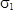) and deviator stress (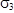)), testing typically is done over a range of confining and deviator stresses. A mathematical model is then fitted to the resilient modulus and confining and deviator stress data for use in estimating resilient modulus for any reasonable combination of confining and deviator stresses.

Developing correlations between resilient modulus and basic unbound granular/coarse-grained and fine-grained materials for use in pavement analysis and design was one of the objectives of this study. As with the other models described in this report, the LTPP database contained adequate data to develop a resilient modulus model for unbound materials.

### Data Assembly for Resilient Modulus Model Development

The literature review included several models developed for the use in predicting resilient modulus using unbound material index properties. From this literature review, a list was developed of all unbound material properties that impact resilient modulus and thus could potentially be included in a resilient modulus prediction model.

Unbound material properties of interest, along with resilient modulus data required for model development as determined through the literature review, were obtained from the LTPP database and assembled in a model development database. Also included in this database were actual resilient modulus test results and the range of confining and deviator stresses at which resilient modulus was determined.

### Assembly of Resilient Modulus Model Development Database

Individual LTPP material database tables were merged to develop the resilient modulus model development database. Because the LTPP database is a relational database (i.e., it is composed of separate but related data tables), data assembly was performed by linking data stored in a simple row/column format in tables using unique primary keys to identify LTPP test sections or projects. For many of the data tables, the primary keys were the combination of STATE_CODE, SHRP_ID, and CONSTRUCTION_NO.

The next step was to determine the AASHTO soil classification for each unbound material of the database. The gradation and Atterberg limits were used to determine the soil classification.

### Data Review

The assembled data were reviewed thoroughly to identify anomalies and missing data elements, as well as to assess the reasonableness of the data. While this review was performed for all models developed, the review was more in-depth for the resilient modulus data because of the sheer size of the database and because of the potential for large model errors for relatively small data discrepancies.

Examples of plots summarizing the assembled data used to assess data reasonableness are presented in figure 235 through figure 247. The data were assembled and reviewed by each AASHTO soil class to assess if the trends in the data were reasonable.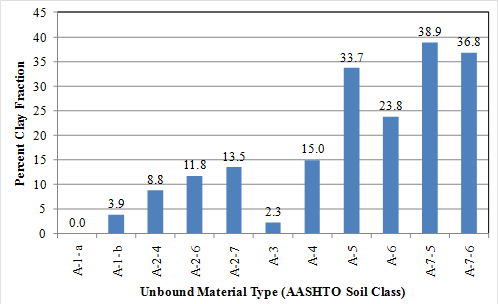#### Figure 235. Graph. Mean percent clay fraction for unbound material types included in the model development database.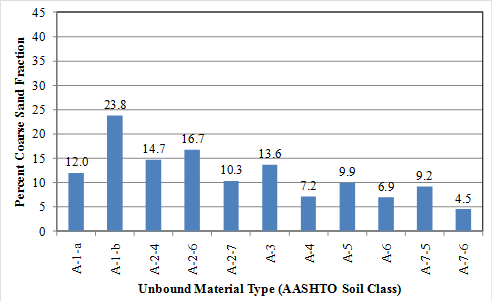#### Figure 236. Graph. Mean percent coarse sand fraction for unbound material types included in the model development database.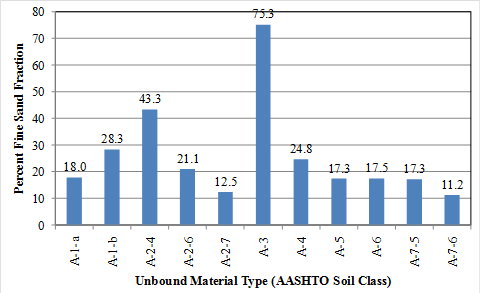#### Figure 237. Graph. Mean percent fine sand fraction for unbound material types included in the model development database.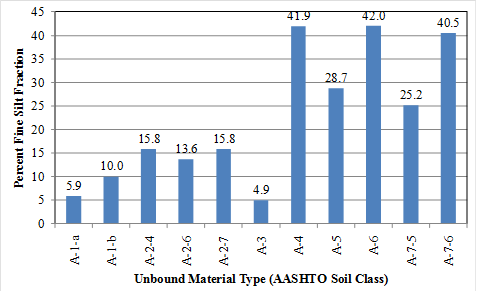#### Figure 238. Graph. Mean percent silt fraction for unbound material types included in the model development database.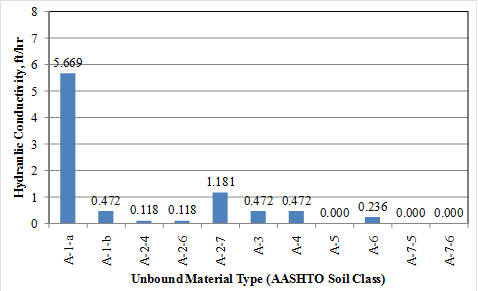#### Figure 239. Graph. Mean hydraulic conductivity for unbound material types included in the model development database.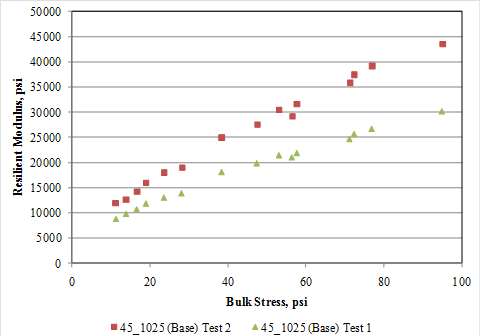#### Figure 240. Graph. Plot of bulk stress versus lab tested resilient modulus for section 45_1025 (base layer).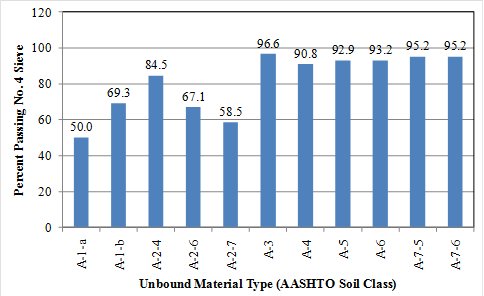#### Figure 241. Graph. Percent passing No. 4 sieve for unbound material types included in the model development database.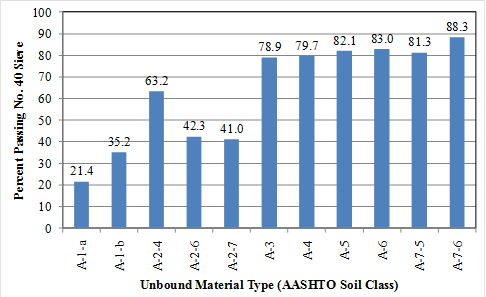#### Figure 242. Graph. Percent passing No. 40 sieve for unbound material types included in the model development database.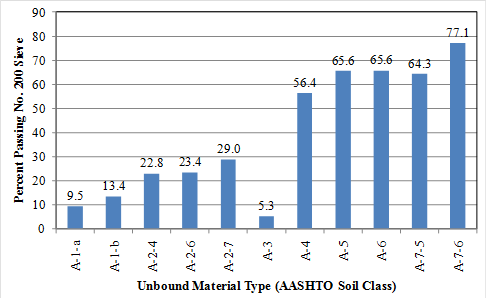#### Figure 243. Graph. Percent passing No. 200 sieve for unbound material types included in the model development database.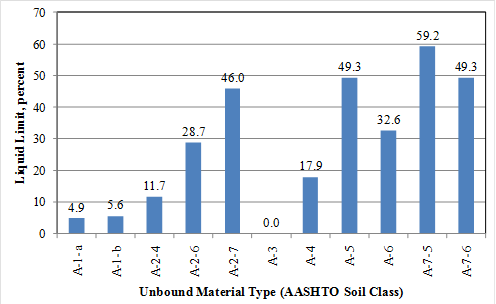#### Figure 244. Graph. Liquid limit for unbound material types included in the model development database.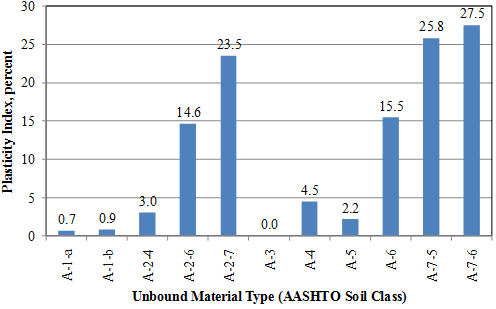#### Figure 245. Graph. Plasticity index for unbound material types included in the model development database.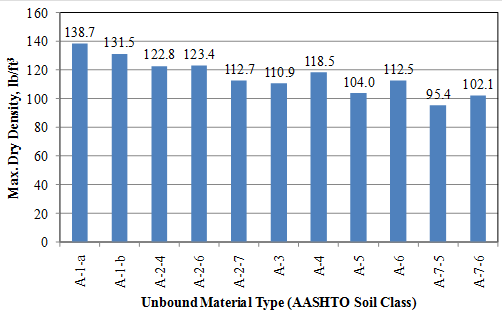#### Figure 246. Graph. Maximum dry density for unbound material types included in the model development database.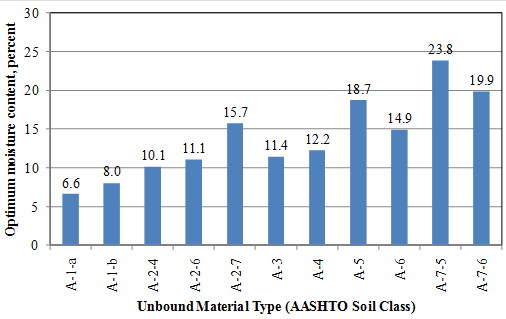#### Figure 247. Graph. Optimum moisture content for unbound material types included in the model development database.

The following conclusions were made based on the review of assembled data:

• All key data elements required for resilient modulus model development were available.
• Data available were of adequate quality and completeness for use in model development.
• Resilient modulus data were available for a range of confining and deviator stresses. Thus, there was no single test result that represented the typical unbound base and subgrade soil stress state within a pavement structure. Note that stress state within the pavement structure varies considerably according to pavement type, layer types and thicknesses, layer modulus, applied truck loading, etc.

The following anomalies were identified when matching material properties data elements to Mr data for subgrade soils:

• Sampling of unbound soil/granular materials for performing resilient modulus tests and for determining other soil properties may have occurred at different depths of the subgrade, depending on how the test sample was obtained (i.e., from test pits, coring/boring, auger pits, etc).
• Highly variable subgrade soils at a given site could lead to significant differences in materials used for different types of testing.
• Lack of uniformity in test materials types could lead to mismatching of resilient modulus and soil properties data.

### Resolving Identified Anomalies

Over half the test sections did not have all the data elements (e.g., hydraulic conductivity) required to fully characterize the pavement subgrade. However, sufficient data were available for key subgrade material properties (e.g., gradation and Atterberg limits). Data elements for which little data were available were not used in model development.

A significant anomaly was differences in subgrade soil materials used for different types of testing due to sampling location. This anomaly was resolved by matching resilient modulus data to other soil test data only when the sample location could be certified as being as close as possible (i.e., same depth/strata, same test pit, etc.).

A second anomaly was the lack of a single representative resilient modulus value for a given unbound material sample (i.e., series of resilient modulus values corresponding to a combination of deviator and confining stresses used during testing process). This situation has been resolved in the past by fitting the series of resilient modulus values corresponding to a combination of deviator and confining stresses used during testing process with a constitutive equation that models resilient modulus behavior for both granular and fine-grained materials.

As defined in chapter 3, the AASHTO Mechanistic-Empirical Pavement Design Guide, Interim Edition: A Manual of Practice proposes the constitutive equation for modeling resilient modulus behavior when subjected to various stress states the following:(1)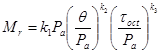#### Figure 248. Equation. Mr.

Where: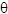= Bulk stress =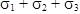.= Principal stress.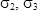= Confining pressure.

Pa = Atmospheric pressure.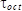= Octahedral normal stress = 1/3 (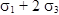).

k1, k2, k3 = Regression constants that are a function of soil properties, as defined in figure 75, through figure 77.

This model can be used for various soil types, and the model attributes (k1, k2, and k3) for a given soil type remain the same regardless of stress state. Developing models to predict constitutive model attributes for a given set of soil properties is thus an effective approach to modeling resilient modulus behavior, rather than developing models individually for each possible combination of expected stress states.

### Estimating Resilient Modulus Constitutive Model Parameters k1, k2, and k3

Figure 33 presents the constitutive equation that models resilient modulus behavior for both granular and fine-grained materials recommended by the AASHTO Mechanistic-Empirical Pavement Design Guide, Interim Edition: A Manual of Practice.(1) The parameters k1, k2, and k3 were calculated for all datasets based on the soil properties. Histograms showing the distribution of k1, k2, and k3 values by soil class are shown in figure 249 through figure 251, respectively.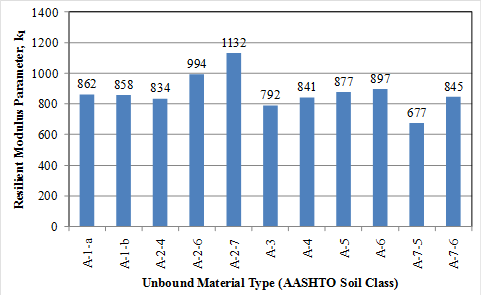#### Figure 249. Graph. Resilient modulus parameter k1 for unbound material types included in the model development database.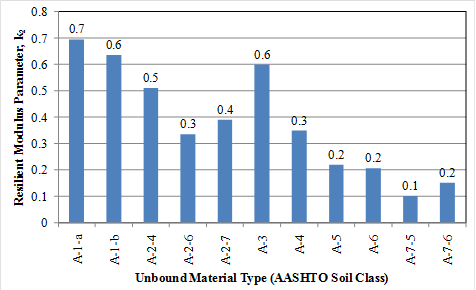#### Figure 250. Graph. Resilient modulus parameter k2 for unbound material types included in the model development database.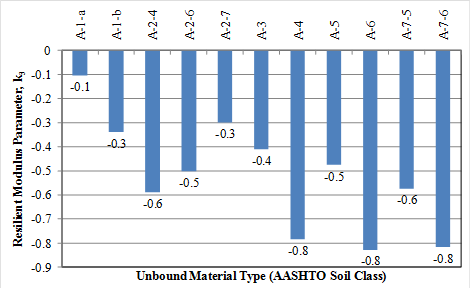#### Figure 251. Graph. Resilient modulus parameter k3 for unbound material types included in the model development database.

This model was fitted to all soil samples with resilient modulus available for a range of confining and deviator stresses. Model fitting was done individually for each unbound material sample in the LTPP database. For each of the soil samples, calculated k1, k2, and k3 and the constitutive equation were used to predict resilient modulus at the lab test confining and deviator stresses for comparison. The results of the comparison showed a good fit of predicted and measured resilient modulus with a high R2 value and low standard error of estimate (SEE), as presented in figure 252.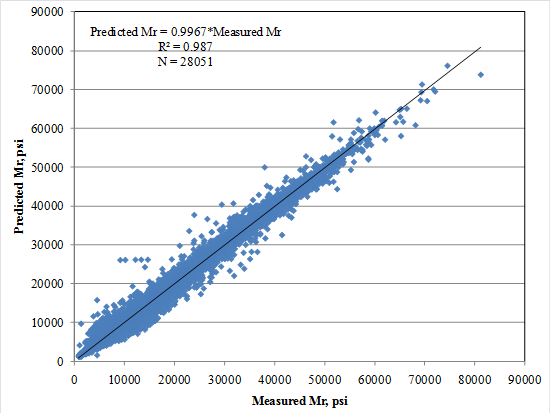### Resilient Modulus Model Development

The following five-step procedure using regression analysis was used to develop multiple linear regression models relating resilient modulus to unbound material properties:

1. Determine appropriate model form.
2. Develop inputs for regression analysis.
3. Perform separate regression analyses for each soil class as follows:
• Partition assembled data for use in actual model development and validation of tentative models.
• Perform regression analysis and develop tentative models.
• Verify tentative model by validating with “set aside” data and checking various model diagnostic statistics to determine the suitability of tentative models developed.
• Select optimal model inputs and establish k1, k2, k3 and material properties relationship.
4. Perform regression with full dataset and determine optimal model coefficients and diagnostic statistics.
5. Perform sensitivity analysis and determine final model coefficients.

Details of the procedure are explained in the following sections.

### Step 1: Select Appropriate k1, k2, k3 Prediction Model Form

Various forms of mathematical relationships have been used for relating constitutive model parameters k1, k2, and k3 to simple material properties. The two most commonly applied, and hence most promising, model forms are as follows:

• ki = f (material properties).
• log(ki) = f (material properties).

Where ki is k1, k2, and k3. Because both of these mathematical equations have been used successfully, they were both deemed appropriate and were adopted for model fitting in this project.

### Step 2: Develop Inputs for Regression Analysis

The data assembled contained all inputs required for model development. Details are as previously described.

### Steps 3 and 4: Perform Regression Analyses

Numerous preliminary multivariable regression runs were performed to produce the “best” model. The goal was to determine the optimal set of independent variables (material properties) that, when included in the model, will maximize adjusted R2 values and minimize errors (SEE). Other diagnostic statistics, such as the level of significance of the regression coefficient of each independent variable, COLLIN (used to check for multicollinearity) in SAS®, and VIF were made to determine the goodness of fit for the model.

Independent variables with regression coefficients significant at the 0.05 significance level (determined through performing t-tests) were retained in the models developed. The t-test threshold was set at this level so that only variables that impacted k1, k2, and k3 were significantly included in the models. The resulting models developed through regression analysis for constitutive model parameters k1, k2, and k3 are described below.

Constitutive Model Parameter k1: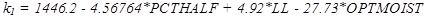#### Figure 253. Equation. Prediction model 18 for k1.

Model statistics for k1 are as follows:

• R2 = 0.16 percent.
• SEE = 237.4.
• N = 1,029.

Constitutive Model Parameter k2: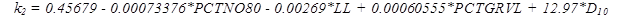#### Figure 254. Equation. Prediction model 19 for k2.

Model statistics for k2 are as follows:

• R2 = 0.67 percent.
• SEE =  0.0934.
• N = 1,032.

Constitutive Model Parameter k3: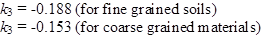#### Figure 255. Equation. Prediction model 20 for k3.

Where:

PCTHALF = Percent passing 1/2-inch sieve.

LL = Liquid limit, percent.

OPTMOIST = Optimum moisture content, percent.

PCTNO80 = Percent passing No. 80 sieve.

PCTGRVL = Percent gravel fraction (0.078- to 2.36-inch size).

D10 = Maximum particle size of the smallest 10 percent of soil sample.

Model prediction accuracy and reasonableness were evaluated by reviewing the plot of predicted and measured resilient modulus for all individual resilient modulus test values used in model development as presented in figure 256. Note that the plot presents actual measured resilient modulus for each individual sample and stress state and resilient modulus computed using predicted k1, k2, and k3 based on actual material properties for each individual sample and the resilient modulus constitutive model and stress state. Figure 257 presents a plot of measured and predicted resilient modulus versus bulk stress for all fine- and coarse-grained materials included in model development database. Note that mean measured k1, k2, and k3 for coarse- and fine-grained materials and predicted k1, k2, and k3 using the equations in figure 70 through figure 72 and mean input values for fine- and coarse-grained materials were used for developing this plot. A review of the plots presented in figure 256 and figure 257 shows a reasonable prediction of resilient modulus.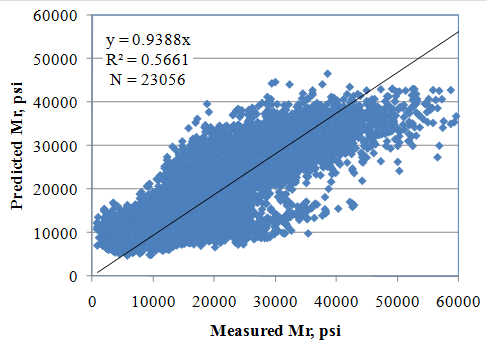#### Figure 256. Graph. Measured versus predicted resilient modulus (using k1, k2, and k3 from figure 70 through figure 72).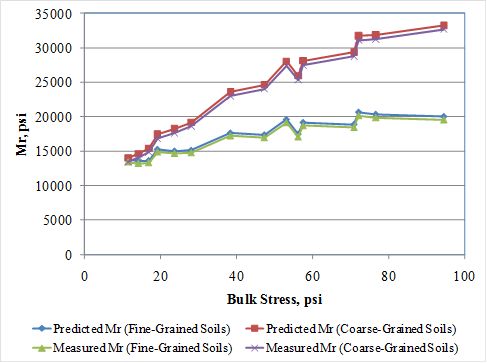### Step 5: Conduct Sensitivity Analysis

The tentative models were further evaluated by conducting a comprehensive sensitivity analysis. The goal was to determine if the model behaves as expected based on engineering principles. Sensitivity analysis results are presented in figure 258 through figure 264. The results of the sensitivity analysis are summarized as follows:

• Soil type had a significant impact on predicted resilient modulus. Coarse-grained materials show significantly higher levels of resilient moduluswith increasing bulk stress.
• Increasing the amount of finer materials resulted in a decrease in resilient modulus.
• Increasing the amount of gravel resulted in increased resilient modulus.
• Increasing effective size increases resilient modulus.
• Increasing optimum moisture content resulted in reduced values of resilient modulus.
• Increasing liquid limit resulted in an increase in resilient modulus.

Overall, the trends observed were deemed reasonable, and the proposed model was established as the recommended model for resilient modulus prediction.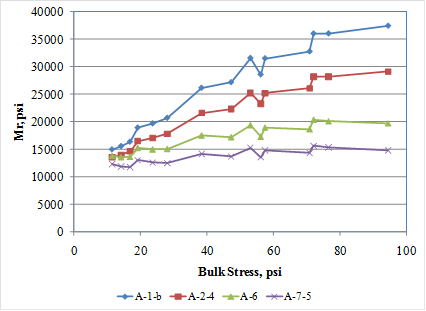#### Figure 258. Graph. Effect of material type (AASHTO soil class) on predicted resilient modulus.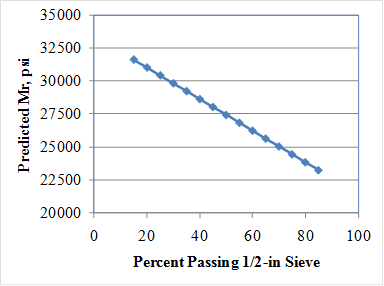#### Figure 259. Graph. Effect of percent passing 0.5-inch sieve on predicted resilient modulus.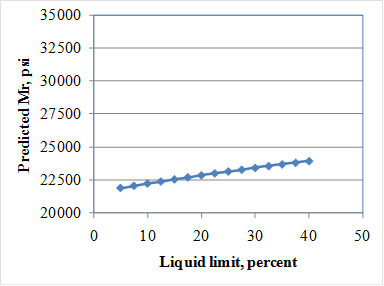#### Figure 260. Graph. Effect of liquid limit on predicted resilient modulus.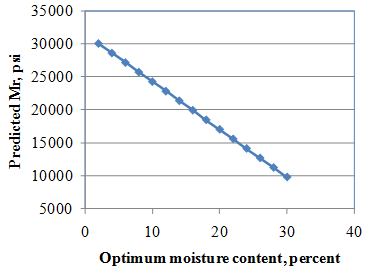#### Figure 261. Graph. Effect of optimum moisture content on predicted resilient modulus.#### Figure 262. Graph. Effect of No. 80 sieve on predicted resilient modulus.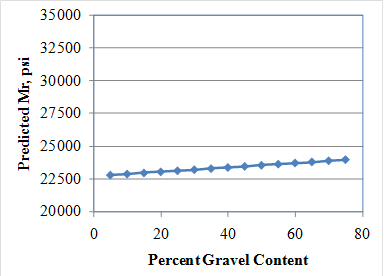#### Figure 263. Graph. Effect of gravel content on predicted resilient modulus.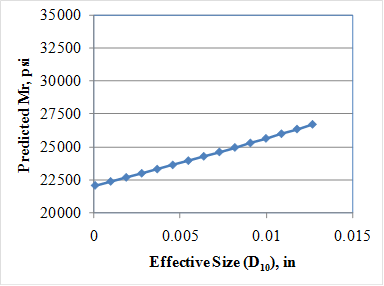## Practical Guide and Software Program

The models developed under this study have been incorporated into a user-friendly software program, Correlations, which can be used independently from the MEPDG. The software, developed under this study, was developed on the Microsoft.NET platform to be compatible with the latest versions of the Microsoft Windows® operating systems. It is programmed in the C# language and uses a modern user interface library to provide a familiar look and feel. It features multiple windows on the user interface that are initially docked inside the main window. These windows can be moved separately from the main window for better viewing of the inputs or results.

The program interface features tabs for PCC, design features, stabilized materials, and unbound materials. Models that belong to each of these categories are made available through a series of radio button selections placed in an accordion control. This placement not only provides the ability to make multiple selections, but it also conserves screen space so that the results of the calculations can be placed for easy viewing. Once a model is selected, the entry area adds controls for the available inputs of the model.

Throughout the software, tooltips are used to provide feedback for each of the areas where data can be entered. Calculations occur after all necessary values have been input. Information about each of the models is available in an information window initially located at the bottom of the screen. This information is context-sensitive to the specific selections that the software user has made. Results of each calculation are displayed prominently in the results area window, initially placed on the right side of the main window.

The software program may be requested from the LTPP Customer Support Service Center at ltppinfo@dot.gov.

Federal Highway Administration | 1200 New Jersey Avenue, SE | Washington, DC 20590 | 202-366-4000
Turner-Fairbank Highway Research Center | 6300 Georgetown Pike | McLean, VA | 22101